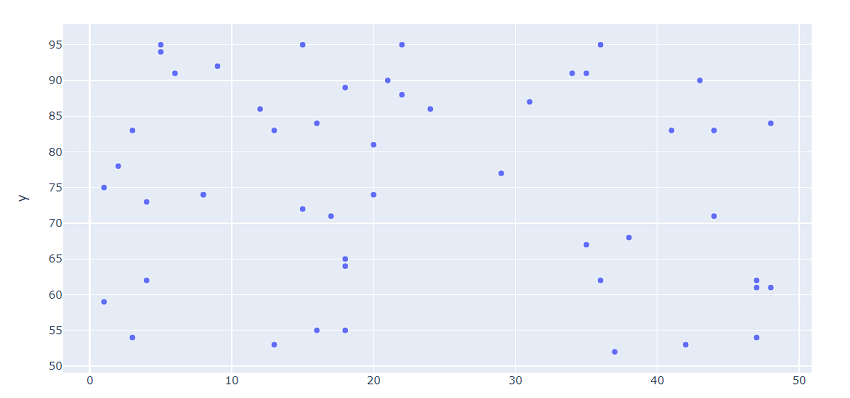# Introduction to Plotly-online using Python

The plotly library is an interactive open-source library. This can be a very helpful tool for data visualization and understanding the data simply and easily. Plotly graph objects are a high-level interface to plotly which are easy to use. It can plot various types of graphs and charts like scatter plots, line charts, bar charts, box plots, histograms, pie charts, etc.

Python is also capable of uploading created graph online for use in websites. In this article, a simple scatter plot is created to be used in websites and this graph is working on the particular website that is the beauty of plotly and chart-studio.

### Installation:

```pip install plotly
pip install chart-studio             ```

### Approach:

Part A: Creating a graph

• Import module
• Create data
• Create plot
• Display graph

Part B: Taking the graph online

• After signing in find your username and API key in the settings section. (go to profile > settings > regenerate key)
• Now in your python script
• Again import module
• Pass API keys and username
• Pass all the necessary information to plot() function
• The output returned is a link, where the graph can be seen live.

Below is the implementation.

## Python3

 `# importing required libraries ` `import` `numpy as np ` `import` `plotly ` `import` `chart_studio ` `import` `plotly.express as px ` ` `  `# assigning values to x and y ` `x ``=` `np.random.randint(low``=``1``, high``=``50``, size``=``50``) ` `y ``=` `np.random.randint(low``=``51``, high``=``100``, size``=``50``) ` ` `  `# creating and displaying graph ` `fig ``=` `px.scatter(x``=``x, y``=``y) ` `fig.show() `

Output:Graph Between X and Y generated in Plotly

Now as our graph is created. It’s time to use it online on websites.

## Python3

 `import` `chart_studio ` `username ``=`  `# 'your username' ` `api_key ``=`  `# 'your api key' ` ` `  `chart_studio.tools.set_credentials_file(username``=``username, api_key``=``api_key) ` ` `  `py.plot(fig, filename``=``'your filename'``, auto_open``=``False``, sharing``=``'public'``) `

Output: